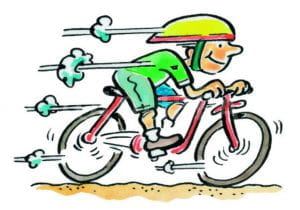## Welcome to H3 Maths

Blog Support for Growing Mathematicians

December13

Most of us have a basic understanding of power. Walking upstairs takes a certain level of effort, but when we add a large sack of potatoes to the shoulder it takes more energy.Power is commonly measured in watts. Variables here but riding a bike at a leisurely 15 mph on a flat windless road is something like 50 watts of effort. Riding at 35 mph would be in the 200 watt range. A horsepower is 745 watts.

An elite cyclist can average 300 watts or more for a few hours. An elite sprinter can hit 1500 watts for 10 seconds or so. You get the idea.

by posted under Uncategorized | No Comments »

#### Post Support

10 x 9 x 8 + (7 + 6) x 5 x 4 x (3 + 2) x 1 = 2020

NCEA Level 2 Algebra Problem. Using the information given, the shaded area = 9, that is:
y(y-8) = 9 –> y.y – 8y – 9 =0
–> (y-9)(y+1) = 0, therefore y = 9 (can’t have a distance of – 1 for the other solution for y)
Using the top and bottom of the rectangle,
x = (y-8)(y+2) = (9-8)(9+2) = 11
but, the left side = (x-4) = 11-4 = 7, but rhs = y+? = 9+?, which is greater than the value of the opp. side??
[I think that the left had side was a mistake and should have read (x+4)?]